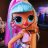# Moving average crossover with volume spike?

P

#### Playstation

##### Active member
VIP
I found this online, but couldn't figure out how to plot an up or down arrows when the crossover with volume spike. Help? Conditions to trigger alert is:

CloseAbove and VolX and strongEup = arrow up
CloseBelow and Volx and strongEdn = arrow down

These are found at the bottom of the script.

Code:
``````# 3 moving averages crossover signals

input SMA_short = 5;
input SMA_medium = 20;
input SMA_long = 50;

def AvgS = Average(close, SMA_short);
def AvgM = Average(close, SMA_medium);
def AvgL = Average(close, SMA_long);
def Candle_White = close > open;
def Candle_Red = close < open;

def Eup = (AvgS > AvgM and AvgM > AvgL);
def Edn = (AvgS < AvgM and AvgM < AvgL);
def CrossUp = Eup and !Eup and close > AvgM;
def CrossDn = Edn and !Edn and close < AvgM;

def BuySignalUp = if (Eup and Candle_White and CrossUp and close > (if Candle_White then close else open)) then 1
else
if (Eup and Candle_White and CrossUp and close < (if Candle_White then close else open) and close > (if Candle_White then close else open)) then 1
else
Double.NaN;

def BuySignalDn = if (Edn and Candle_Red and CrossDn and close < (if Candle_Red then close else open)) then 1
else
if (Edn and Candle_Red and CrossDn and close > (if Candle_Red then close else open) and close < (if Candle_Red then close else open)) then 1
else
Double.NaN;

def strongEup = Eup and close > AvgS;
def strongEdn = Edn and close < AvgS;

plot bullish = Long;
bullish.SetPaintingStrategy(PaintingStrategy.BOOLEAN_ARROW_UP);
bullish.SetDefaultColor(Color.CYAN);
bullish.SetLineWeight(3);

plot Bearish = Short;
Bearish.SetPaintingStrategy(PaintingStrategy.BOOLEAN_ARROW_Down);
Bearish.SetDefaultColor(Color.CYAN);
Bearish.SetLineWeight(3);

#VOLUME SPIKE
input AverageLength = 10;
input VolumeMultiplier = 3;
input MinutesAfterOpen = 1;

def AvgVol = average(volume, AverageLength);
def VolX = (volume > AvgVol * VolumeMultiplier) and (secondsfromtime(0930) >= 60 * MinutesAfterOpen);

#Out of Bounds
def sDev = StDev(close, 21);
def MidLine = Average(close, 21);
def UpperBand = MidLine + 2 * sDev;
def LowerBand = MidLine - 2 * sDev;
def CloseAbove = if close > UpperBand then 1 else Double.NaN;
def CloseBelow = if close < LowerBand then 1 else Double.NaN;

Alert(CloseAbove and VolX and strongEup , Concat(GetSymbolPart(), " Above Upper Band." ), Alert.BAR, Sound.Bell);

Alert(CloseBelow and Volx and strongEdn , Concat(GetSymbolPart(), " Below Lower Band" ), Alert.BAR, Sound.Bell);``````

Last edited by a moderator:Simple moving average crossover scan Questions 1